International
Tables for
Crystallography
Volume B
Reciprocal space
Edited by U. Shmueli

International Tables for Crystallography (2006). Vol. B. ch. 2.1, pp. 196-197   | 1 | 2 |

## Section 2.1.5.6. Cumulative distribution functions

U. Shmuelia* and A. J. C. Wilsonb

aSchool of Chemistry, Tel Aviv University, Tel Aviv 69 978, Israel, and bSt John's College, Cambridge, England
Correspondence e-mail:  ushmueli@post.tau.ac.il

#### 2.1.5.6. Cumulative distribution functions

| top | pdf |

The integral of the probability density functionfrom the lower end of its range up to an arbitrary value x is called the cumulative probability distribution, or simply the distribution function,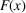, of x. It can always be written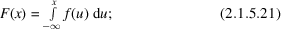if the lower end of its range is not actually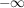one takesas identically zero betweenand the lower end of its range. For the distribution of A [equation (2.1.5.4)or (2.1.5.9)] the lower limit is in fact; for the distribution of,, I and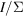the lower end of the range is zero. In such cases, equation (2.1.5.21)becomes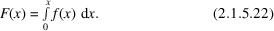In crystallographic applications the cumulative distribution is usually denoted by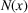, rather than by the capital letter corresponding to the probability density function designation. The cumulative forms of the ideal acentric and centric distributions (Howells et al., 1950) have found many applications. For the acentric distribution of[equation (2.1.5.8)] the integration is readily carried out: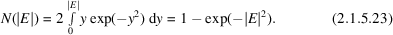The integral for the centric distribution of[equation (2.1.5.11)] cannot be expressed in terms of elementary functions, but the integral required has so many important applications in statistics that it has been given a special name and symbol, the error function erf(x), defined by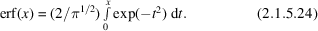For the centric distribution, then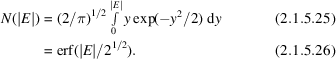The error function is extensively tabulated [see e.g. Abramowitz & Stegun (1972), pp. 310–311, and a closely related function on pp. 966–973].

### ReferencesAbramowitz, M. & Stegun, I. A. (1972). Handbook of mathematical functions. New York: Dover.Google ScholarHowells, E. R., Phillips, D. C. & Rogers, D. (1950). The probability distribution of X-ray intensities. II. Experimental investigation and the X-ray detection of centers of symmetry. Acta Cryst. 3, 210–214.Google Scholar